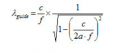# group wavelength in coax to waveguide transition calculation went wrong

#### yef smith

Joined Aug 2, 2020
397
Hello,We excite the coax input by some freequency in CST simulation.
i need to locate the coax at 0.25*lambda_g from waveguide wall.
so if mycoax input is at f=2.4G i have tried to input the waveguide dimention as shown bellow in matlab.
matlab gives me complex number ( 0.0000 - 0.1677i) in the result.
Where did i go wrong?
Thanks.
Code:
c=3*10^8;
f=2.4*10^9;
a=98.06*10^-3;

sqt=sqrt(1-pow2(c/(2*a*f)))
formula=(c/f)*(1/sqt)Last edited: